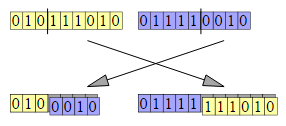# Eggs aren't vegIf every letter corresponds a unique digit in decimal system and $\overline{TOM}+\overline{GIVES} = \overline{MOST}+ \overline{TIME}$ Find the value of $\overline{EGG}+ \overline{IS} + \overline{VEG}$

Details and assumptions:

$\bullet$ If $(A,B,C,D) =(1,2,3,4)$ , then $\overline{BAD} = 214$.

$\bullet$ Everything is in decimal representation and every letter corresponds to one unique digit. That is why this has exactly one solution.

Everything that i shared on brilliant

×